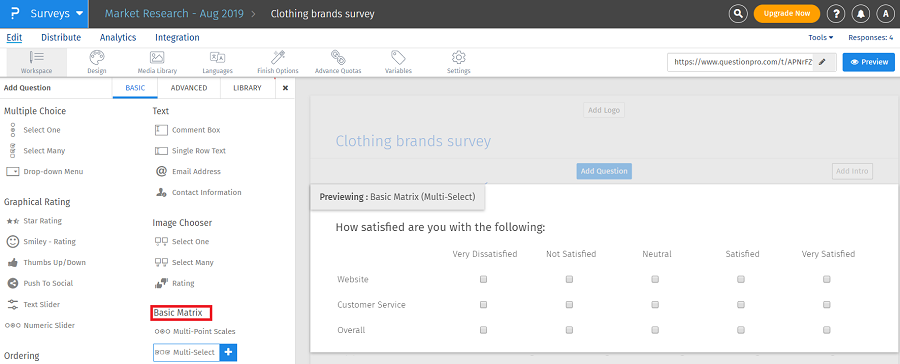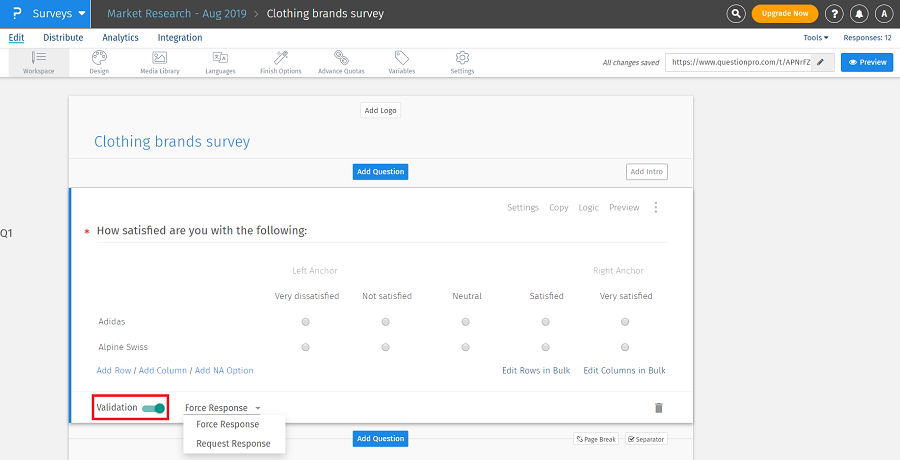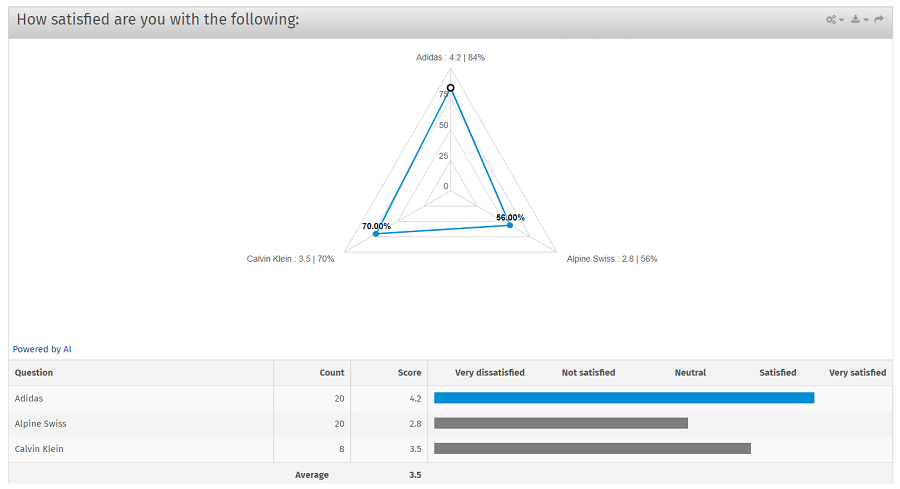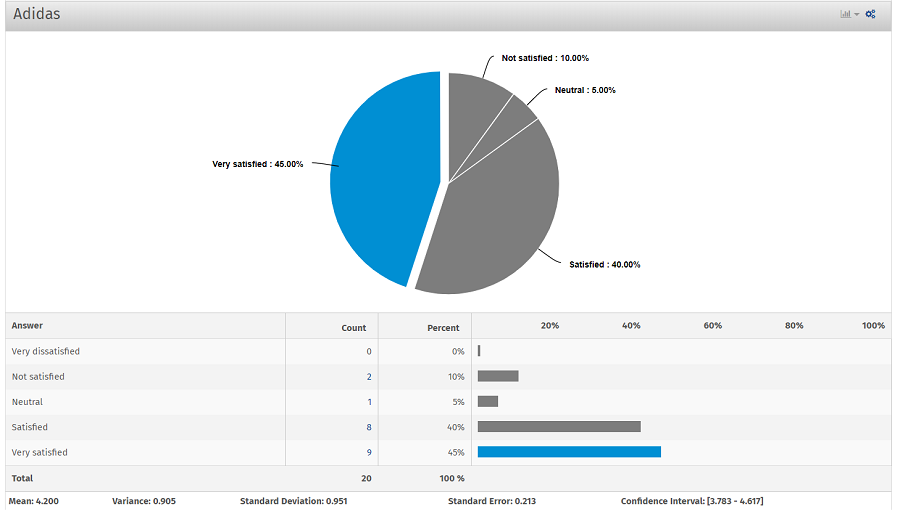# Matrix multi-select question

The matrix multi-select question allows respondents to choose multiple answer options for each row in the matrix table. You can use matrix multi-select question to group various individual multi-select questions that share the same answer options.

## How do I add matrix multi-select questions?

• Go to: Login » Surveys (select a survey) » Edit » Workspace
• Click on the add question button.
• Under basic question types » basic matrix, click on multi-select question.
•• Matrix multi-select question appears.
•• Edit rows in bulk: This option helps to add row choices in bulk. We can copy and paste the list of answer options directly in this field.
• Edit columns in bulk: This option helps to add column choices in bulk. We can copy and paste the list of answer options directly in this field. You can also enable the NA option from this field.
• Validation: When you add questions to a survey, by default, validation option is turned off. When validation option is not enabled, respondents can continue with the survey without selecting answers. If respondents go through all the pages in the survey without selecting answers, the response is still considered as complete. By enabling validation option you can see a drop-down with options - force response and request response.

Force response option makes a question mandatory/required, as shown in the above image.

When you select request response, the respondent gets a pop-up in case a question is not answered, requesting to answer the question or continue survey without answering.

• Select at most/exactly/at least: You can put validation on number of choices which a respondent must select before proceeding to the next question. For example, if you want the respondent to select maximum 2 answer options then choose select at most from the drop down and set the value as 2.

## How do I view the report for matrix multi-select question?

To view the report for matrix multi-select question:

• Go to: Login » Surveys (select a survey) » Analytics » Dashboard.

You can view the matrix multi-select question in the form of spider-chart:

•• In the spider-chart shown above, you can see score (mean) for each question/row and percentage (%) of the score.
• Score: This is the mean value calculated for the responses of each question (row) under matrix questions.
• Percentage (%): This is the percentage calculated for the score of each question.

Formula: Percentage = Score/No. of options * 100. For example, the score for Adidas is 4.2 and no. of options are 5. Hence, the percentage for Adidas is 84% (4.2/5 * 100).

• Since matrix multi-select question is a group of individual multi-select questions, individual reports for each row are also diplayed below spider-chart. For example, the report for Adidas is displayed as follows:
•• Similarly, the reports for Alpine Swiss and Calvin Klein are also displayed.
• You can download the report in Excel or CSV format from the dashboard.